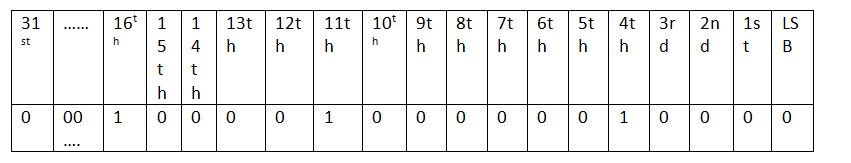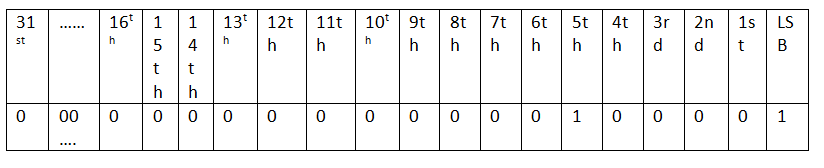# C program to Check if nth Bit in a 32-bit Integer is set or not

In this article, we are going to see how to find nth bit of a 32-bit integer is set or not using bitwise operator?
Submitted by Radib Kar, on January 06, 2019

Problem statement: Write a C program to check if nth bit is set or not in a 32 bit integer.

Solution: Pre-requisite: input no(32 bit longer), nth bit

Algorithm

1. Right shift by n times to get the nth bit at LSB
2. Do a bitwise and with 1(only LSB is set of 1, other bits 0).
3. IF result is 1, then nth bit is set
Else
Bit not set

Example with explanation:

Let input no be 67600 and n is 11This is the binary representation of 67600

After right shifting 11 times,So bitwise ANDing this shifted no with 1

Results in 00000...0001(31 0's & one 1) which is 1... Thus n th bit is set...

## C implementation to Check if nth Bit in a 32-bit Integer is set or not

```#include <stdio.h>

int main()
{
int n,k;
printf("enter a 32 bit number\n");
scanf("%d",&k);
printf("enter the bit no to check...\n");
printf("bit-no 0-indexed & 0 starts from LSB...\n");
scanf("%d",&n);
if(n>32){
printf("enter between 0-31\n");
return;
}

k=k>>n; //right shift the no to get nth bit at LSB
if(k&1==1) //check whether nth bit set or not
printf("%d th bit is set\n",n);
else
printf("%d th bit not set\n",n);

return 0;

}
```

Output

```First run:
enter a 32 bit number
67600
enter the bit no to check...
bitno 0-indexed & 0 starts from LSB...
11
11 th bit is set

Second run:
enter a 32 bit number
67600
enter the bit no to check...
bit-no 0-indexed & 0 starts from LSB...
10
10 th bit not set
```